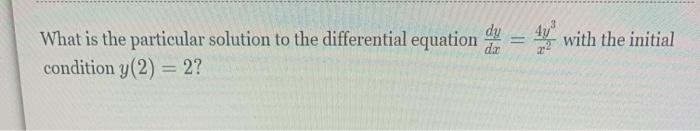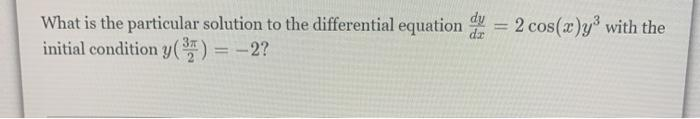# Question with the initial dy What is the particular solution to the differential equation condition y(2) = 2? dac What is the particular solution to the differential equation og = 2 cos(x)y with the initial condition y() = –2?